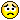0
|
2127 Views
|
4 Replies
|
2 Total Likes
View groups...
Share
GROUPS:

# How to Plot an Inequality

Posted 10 years ago
 Hello everyone!I would kindly need some help for this:How can I plot in mathematica the graphic solution for: -(T+q) z + (1-T-q) r  <  -z / 2 ?where T and q are probabilities 00 and z>0 positive parameters          I tried:  ParametricPlot3D[-(T + q) z + (1 - T - q) r < -(z / 2) && 0 < T < 1 &&0 < q < 1, && r > 0 && z > 0, {B, 0, 1}, {p, 0, 1}]But doesn't work.. :/
4 Replies
Sort By:
Posted 10 years ago
 Oh, ok, I got it!..sorryThanks a lot David!
Posted 10 years ago
 Hi Kodi,Perhaps I don't understand. The code block I posted produces this result:Posted 10 years agoI still can't get it(Also, I think I would need the conditions of the paramters (>0) and the probabilities)
Posted 10 years ago
 RegionPlot:plotRegion[r_, z_] := RegionPlot[-(t + q) z + (1 - t - q) r < -z/2, {t, 0, 1}, {q, 0, 1},   FrameLabel -> {"t", "q"}, PlotLabel -> "-(t+q) z+(1-t-q) r<-z/2"]Best regards,David